### 4.1 Exposure

As explained in the previous module, when radiation interacts with matter, electrons are removed from atoms through the process called ionization. When the energy of the radiation is not strong enough, electron excitation (jumps from lower shells to upper shells) may occur. The excitation of molecules or breaking of molecular bonds can also occur, causing damage to living cells. When the energy of radiation is large enough to produce ionization, the radiation is called ionizing radiation. Otherwise, it is called non-ionizing radiation.

When dealing with the interaction of gamma and X-rays in air, the term exposure is used. Exposure measures the electric charge (positive or negative) produced by electromagnetic radiation in a unit mass of air, at normal atmospheric conditions.

In the SI system of units, exposure is measured in X unit: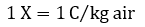where C is Coulomb, the SI unit for electric charge.

The average energy dissipated to produce a single ion pair in air is 34 eV. Since the charge of an electron is equal to 1.6 x 10-19 C, an association can be established between an X unit and the energy measured in Joules, dissipated in 1kg of air.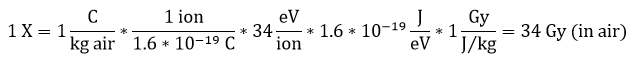Hence, one X unit is equivalent to 34 J/kg.

Formerly, before the SI system was adopted, the unit of X-ray exposure was called the Roentgen (R). R was defined as the quantity of gamma or X-radiation that can produce ions carrying one statcoulomb (1 statC = 3.335 * 10-10 C) of charge (of either sign), per cubic centimetre of air at 0 degrees Celsius and 760 mm Hg of pressure (1 atm).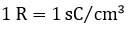The statCoulomb is the old unit of measure for electric charge. The relation between SI unit (C) and statC is 1C = 3 x 109 statC. As mentioned above to produce an ion in air (electric charge of 1.6 x 10-19 C) an average energy of 34 eV = 34 x 1.6 x 10-19 J is required. Since the mass of one cubic centimetre of standard air is 0.001293 g, an exposure of 1 Roentgen corresponds to absorbing energy of 0.00877 J/kg in dry air.

With the above associations we can now establish the correspondence between X unit and R: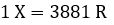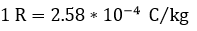At a distance “d” from a point source of radiation, exposure follows an inverse square law, that is, when the distance increases by a factor “k”, exposure decreases by a factor “k2”).

#### Example:

Exposure from a Cs-137 point source at 10 cm is 10 R. Find the exposure at 1 m.

The distance between the Cs-137 source and the point at which exposure is determined to have increased 10 times. Therefore, the exposure at 1 m will be 100 (102) times less, i.e. 0.1 R = 100 mR.
Exposure is a very useful physical term because it can easily be measured. We just have to measure the charge produced by the radiation in air (or the electrical current, multiplied by time) to find out the strength of the electromagnetic field. The instruments used for this purpose are ionization chambers. Measurement results are usually expressed in R or mR.

### 4.2 Absorbed Dose

Although exposure from gamma or X-rays is easily measured in everyday life, we also deal with other types of radiation, like alpha, beta, or neutron. Additionally, we are also interested in the effects of radiation in other materials (like human tissue, for example) not only in dry air.

To measure the interaction of all types of radiation with any kind of material, the term absorbed dose is used. The units of measure for absorbed dose in the SI system is J/kg or gray (Gy). Named after Louis Harold Gray. A radiation field that deposits 1 Joule of energy in 1 kg of material has an absorbed dose of 1 Gy.The old unit of measure for absorbed dose is rad (short for “radiation absorbed dose”). The relationship between the SI unit and the old unit is: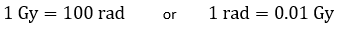There exists a relationship between absorbed dose in dry air and exposure.

As explained above, 1 X unit is equivalent to 34 J/kg or 34 Gy, and 1R = 1X / 3881 = (34 Gy) / 3881.

Therefore: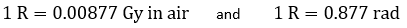However, a more important relationship exists between absorbed dose and exposure in tissue (gamma or X radiation). For a standard composition of muscle tissue, with mass and electronic density compared to standard dry air mass and electronic density, we have: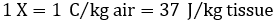For muscle tissue, a good “rule of the thumb” is obtained by combining the above formulae: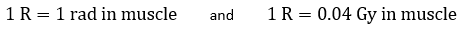Important deviations from this rule appear, especially at low energy, for gamma and X-rays in other types of tissues. Absorbed dose in bone tissue at low energy is more than in muscle, while it is less in adipose tissue.

### 4.3 Equivalent Dose (H)

The term “dose” is used in connection with many other terms. But the most important meaning of dose is connected with radiation stochastic effects. The equivalent and effective dose are used for radiation protection purposes, engineering design criteria and for legal and administrative purposes. Because of this, equivalent and effective dose, in day to day life, are often simply called “dose”.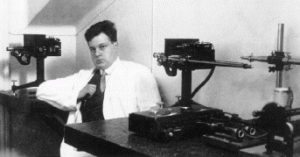Rolf Sievert in his laboratory 1929.

The equivalent dose is defined as: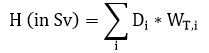Where Di is the absorbed dose for the radiation type “i” (alpha, beta, gamma, x-ray, neutrons, protons, etc.), and WT,i is the radiation weighting factor for the “i” type of radiation. WT,i varies from 1 (for gamma, X-ray and beta radiation) to 20 (for alpha radiation or fission fragments). The old unit for measuring radiation equivalent dose is the rem (short for roentgen equivalent man).

The relationship between exposure, absorbed dose, and equivalent dose is simple for gamma or X-rays when applied to muscle tissue (good approximation).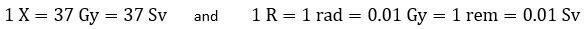N.B.: This “rule of the thumb” can only be applied to gamma and X-rays for muscle tissue. For other types of radiation, or other tissues, the relationship may be very different (e.g. results differ by a factor of 20 for alpha radiation).

### 4.4 Effective Dose

The stochastic effects of ionizing radiation on the whole body are measured with a unit called effective dose. When different organs and tissues are irradiated, the effect on the whole-body is calculated with the formula: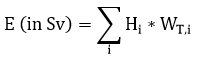Where E is the effective dose, Hi is the equivalent dose of organ or tissue “i” and WTi is the tissue weighting factor. The tissue weighting factor varies between 12% and 1% depending on the sensitivity of each organ to ionizing radiation. The effective dose is also measured in Sv.

### 4.5 External Dose

When the source of radiation is situated outside of the body, the irradiated person will receive what is referred to as an external dose. The calculation of external dose can be performed for the whole body, for extremities, and for superficial skin dose (0.07cm depth) or shallow dose (1cm depth). The type of radiation also plays an important role in assessing the external dose.

For gamma and X-rays, the “inverse square law” can be applied for point sources and absorption in air can be neglected for relatively short distances. For beta radiation, the absorption in air can be very important (depending on the beta energy). For alpha radiation, the external dose is zero since alpha particles cannot penetrate past the layer of dead skin (see Module 3).

Film badges or thermoluminescent dosimeters (TLD) are used to measure the external dose

### 4.6 Internal Dose

Internally deposited radionuclides will irradiate a person’s body from the inside. Typical routes for internal irradiation are inhalation or ingestion of radionuclides and are referred to as intake.

However, radioactive materials are not completely retained in the body. The amount that is retained after an intake is called the uptake. Radionuclides can be retained for varying amounts of time inside the human body. Some are fixed (like Sr-90 inside the bones or I-131 and I-125 inside the thyroid) but the majority of an intake gets eliminated. Therefore, all radionuclides have a “biological half-life”, which is the time after which the uptake decays to half of its original value. This biological half-life is considered in addition to the natural disintegration half-life of the radionuclide.

To most accurately estimate the internal dose, the percent of uptake, biological and natural half-life of the radionuclides, as well as the type of radiation, radiation energy, and preferred organ must be taken into consideration.

### 4.7 Committed dose

After an intake radioisotopes present in the body can irradiate the internal organs for many years to come. The time that each radioisotope is present in the body depends on the physical and biological half-life, the organ, the chemical composition, etc. The equivalent dose resulted from the exposure of a certain organ for the next 50 years (or to age 70 years for children) is called a committed equivalent dose. Similarly, the effects of exposure to the whole body for the next 50 years is called committed effective dose. In a practical application for radioisotopes with a short half-life, the period may be shorter.

### 4.8 Annual Limit of Intake (ALI)

Starting from the permitted dose, an annual limit of intake (ALI) can be calculated for each radionuclide. In Canada, the ALI is defined as the activity (measured in Bq) of a radionuclide that will deliver a committed effective dose of 20 mSv. The ALI values vary with the radionuclide, chemical composition, route of entry in the body, etc. The ALI values are also used in the classification of radioisotope laboratories.

### 4.9 Natural dose

Due to the presence of numerous sources of ionizing radiation in the natural environment (i.e. around us and inside us), the dose received from these types of sources has been named the natural dose, or background.
The natural dose varies around the world by more than a factor of ten. This variation arises from differences in soil composition, type of materials used for building, food and water, to the altitude (the higher the altitude, the larger the irradiation from cosmic sources), etc. Nevertheless, the main contribution (over 50%) comes from the inhalation of radioactive Radon gas products found in the air we breathe. Another important contributor to the natural dose is from K-40 inside our bodies. In North America, the natural dose varies between 2 and 3 mSv (200 and 300 mrem) per year.

### 4.10 Artificial dose

The total dose received by an average person in North America is, in fact, bigger than the amount shown above. This is due to artificially produced radiation and is referred to as the artificial dose. The main artificial dose in North America comes from X-ray machines used in medical diagnoses.

Canadian federal regulations impose a limit for artificial dose received from sources other than medical purposes. This value was recently established for members of the general public at 1 mSv (100 mrem) per year.

### 4.11 Dose rates

Each of the terms used above (Exposure, Absorbed Dose, Radiation Equivalent Dose, etc.) can also be described as a function of time. When doing so, the terms are called: Exposure rate, Absorbed Dose rate, Equivalent Dose rate, etc. The units of measure remain the same but, the values are divided by the time interval. For example, we can have absorbed dose rate received in a minute is measured in mGy/min, effective dose received in a year in mSv/year, etc.